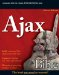# Interpolating Variables in Strings

As discussed earlier in this chapter, you can display the values of variables like this in PHP:

` \$sandwiches = 1; echo "Number of sandwiches: ", \$sandwiches, ".";`

However, there’s a shortcut that you can use here. The values in variables are interpolated if you put them into double-quoted (not single-quoted) strings, which means that their values are inserted directly into the string. You can do that this way to convert the example from the previous code:

` \$sandwiches = 1; echo "Number of sandwiches: \$sandwiches.";`

As you’d expect, this example displays Number of sandwiches: 1.

Here’s a more complete example of variable interpolation, interpolation.php:

` <html>     <head>         <title>             Interpolating variables with PHP         </title>     </head>     <body>         <h1>             Interpolating variables with PHP         </h1>         <?             echo "Setting number of sandwiches to 2.<br>";             \$sandwiches = 2;             echo "Number of sandwiches: \$sandwiches <BR>";             echo "Adding 2 more sandwiches.<BR>";             \$sandwiches = \$sandwiches + 2;             echo "Number of sandwiches now: \$sandwiches <BR>";         ?>     </body> </html>`

You can see the results in Figure 12.6.Figure 12.6: Using interpolation

Interpolating can be useful, but it should be used with caution. What if you wanted to interpolate a variable named \$data containing the word hot to the text dog? That might look like this:

` <?      \$data = "hot";      echo "Want a \$datadog? <br>"; ?>`

This is not going to work, however, because PHP is going to start looking for a variable named \$datadog, as you see here:

` PHP Notice: Undefined variable:   datadog in hotdog.php on line 3`

Instead, the way to do this is to enclose the variable you’re interpolating, \$text, in curly braces, { and }, like this:

` <html>     <head>         <title>             Interpolating variables with PHP         </title>     </head>     <body>         <h1>             Interpolating variables with PHP         </h1>         <?             \$data = "hot";             echo "Want a \${data}dog? <br>";         ?>     </body> </html>`

You do indeed get Want a hotdog? from this example. In general, variable interpolation is a handy shortcut, and one you’ll see often in PHP.Ajax Bible
ISBN: 0470102632
EAN: 2147483647
Year: 2004
Pages: 169

Similar book on Amazon